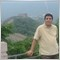# How to Reset a varible - HELP98

Hi.

I'm very new to coding in general so i think this would be an easy fix if.

My problem is that when I run my EA it does all i want until i tell the EA to remove all objects and after that I want it to "reset" it self or to start taking in new values as it dose when I first run it.

Now when it ads my objects to the scen again it dose it at the right time but with all the old values.

How can I fix this?

// Mathias

```//+------------------------------------------------------------------+
//|                                                         test.mq4 |
//|                        Copyright 2015, MetaQuotes Software Corp. |
//|                                             https://www.mql5.com |
//+------------------------------------------------------------------+
#property version   "1.00"
#property strict

//+------------------------------------------------------------------+
//|                        Variables                                 |
//+------------------------------------------------------------------+

int      TimeA=6;
int      TimeB=7;
int      StartHour=TimeA - Hour()*-1;
int      ObjectHour1=StartHour-1;
int      ObjectHour2=StartHour+1;

int      objcount=0;
int      ObjectTime=Time[StartHour];
int      RectangleTime1=Time[ObjectHour1];
int      RectangleTime2=Time[ObjectHour2];
int      ObjRectangle=true;
int      ObjiLow=true;
int      ObjiHigh=true;
int      ObjSell=true;
int      ObjSellBreakeven=true;

double   SumOfPip=0;
double   pip=1234;
double   HighValue=iHigh(0,PERIOD_H1,StartHour);
double   LowValue=iLow(0,PERIOD_H1,StartHour);
//+------------------------------------------------------------------+
//|                           END Variables                          |
//+------------------------------------------------------------------+
//+------------------------------------------------------------------+
//+------------------------------------------------------------------+
//+------------------------------------------------------------------+
//+------------------------------------------------------------------+
//+------------------------------------------------------------------+
//+------------------------------------------------------------------+
//|                        START Function                            |
//+------------------------------------------------------------------+

int start()
{

if(Hour()>=TimeA && Hour()<TimeB)
{
process();

}

//+------------------------------------------------------------------+
if(Hour()==23)
{
ObjectsDeleteAll();

//---------------
ObjRectangle=true;
ObjiLow=true;
ObjiHigh=true;
ObjSell=true;
ObjSellBreakeven=true;
//--------------

}
//+------------------------------------------------------------------+
return(0);
}
//+------------------------------------------------------------------+
//|                       END START Function                         |
//+------------------------------------------------------------------+
//+------------------------------------------------------------------+
//+------------------------------------------------------------------+
//+------------------------------------------------------------------+
//+------------------------------------------------------------------+
//+------------------------------------------------------------------+
//+------------------------------------------------------------------+
//|      Entery Point + Ad Rectangle around Chose TimeCandle         |
//+------------------------------------------------------------------+

int process(){

double SumOfHighValueLowValue=DoubleToStr(HighValue - LowValue, Digits);

SumOfPip=SumOfHighValueLowValue;
pip=DoubleToStr(Close, Digits);
function_2();

//--- Rectangle object
//+------------------------------------------------------------------+
if(ObjRectangle==true){
ObjectCreate("TimeCandle",OBJ_RECTANGLE,0,RectangleTime1,HighValue,RectangleTime2,LowValue);
ObjectCreate("TimeCandle_Text",OBJ_TEXT,0,ObjectTime,LowValue);

ObjectSet("TimeCandle",OBJPROP_COLOR,Red);
ObjectSet("TimeCandle",OBJPROP_WIDTH,1);

ObjectSetText("TimeCandle_Text","TimeCandle",15,"Times New Roman",Red);

ObjRectangle=false;

objcount++;
}
//--- Line at TimeCandle iLow
//+------------------------------------------------------------------+
if(ObjiLow==true){
ObjectCreate("TimeCandle_Low",OBJ_HLINE,0,ObjectTime,LowValue,0,0);
ObjectCreate("TimeCandle_Low_Text",OBJ_TEXT,0,Time,LowValue);

ObjectSet("TimeCandle_Low",OBJPROP_COLOR,Red);
ObjectSetText("TimeCandle_Low_Text","iLow "+LowValue,15,"Times New Roman",Red);
ObjiLow=false;

objcount++;
}
//+------------------------------------------------------------------+
if(ObjiHigh==true){
ObjectCreate("TimeCandle_High",OBJ_HLINE,0,ObjectTime,HighValue,0,0);
ObjectCreate("TimeCandle_High_Text",OBJ_TEXT,0,Time,HighValue);

ObjectSet("TimeCandle_High",OBJPROP_COLOR,Red);
ObjectSetText("TimeCandle_High_Text","iHigh "+HighValue,15,"Times New Roman",Red);
ObjiHigh=false;

objcount++;
}
//+------------------------------------------------------------------+

return(0);
}
//+------------------------------------------------------------------+
//|   END "Process"                                                  |
//+------------------------------------------------------------------+

//+------------------------------------------------------------------+
//+------------------------------------------------------------------+

int function_2(){

double   SellValue_1=LowValue-SumOfPip;
double   SellValue_Breakeven=LowValue-SumOfPip-SumOfPip;

//+------------------------------------------------------------------+

objcount++;
}
//+------------------------------------------------------------------+

objcount++;
}

//--- SellTriggerLine Object
//+------------------------------------------------------------------+
if(ObjSell==true){
ObjectCreate("Sell_Line",OBJ_HLINE,0,ObjectTime,SellValue_1,0,0);
ObjectCreate("Sell_Line_Text",OBJ_TEXT,0,ObjectTime,SellValue_1);

ObjectSet("Sell_Line",OBJPROP_COLOR,Green);
ObjectSet("Sell_Line",OBJPROP_WIDTH,3);
ObjectSetText("Sell_Line_Text","SellTriggerLine",15,"Times New Roman",Green);
ObjSell=false;

objcount++;
}

//--- SellBreakevenTriggerLine Object
//+------------------------------------------------------------------+
if(ObjSellBreakeven==true){
ObjectCreate("SellBreakeven_Line",OBJ_HLINE,0,ObjectTime,SellValue_Breakeven,0,0);
ObjectCreate("SellBreakeven_Line_Text",OBJ_TEXT,0,ObjectTime,SellValue_Breakeven);

ObjectSet("SellBreakeven_Line",OBJPROP_COLOR,Green);
ObjectSet("SellBreakeven_Line",OBJPROP_WIDTH,5);
ObjectSetText("SellBreakeven_Line_Text","SellBreakevenTriggerLine",15,"Times New Roman",Green);
ObjSellBreakeven=false;

objcount++;
}
//+------------------------------------------------------------------+

return(0);
}
//+------------------------------------------------------------------+
```1544

Define your variables as Global (before start( ) ) ,,,

then inside init( ) function use the initial values for those variables.

Then at the end of closeAll( ) function, call init( ) again ... That's all.

By the way, the used time filter will have a problem if TimeB > TimeA ...

It is better to overcome this by using ...

`if((Hour()>=StartHour && Hour()<EndHour && StartHour<EndHour) || ((Hour()>=StartHour || Hour()<EndHour) && StartHour>EndHour))`

Where StartHour = TimeA and EndHour = TimeB

Good luck98

Osama Shaban:

Define your variables as Global (before start( ) ) ,,,

then inside init( ) function use the initial values for those variables.

Then at the end of closeAll( ) function, call init( ) again ... That's all.

By the way, the used time filter will have a problem if TimeB > TimeA ...

It is better to overcome this by using ...

Where StartHour = TimeA and EndHour = TimeB

Good luck

Thank you for the help. This did help me with some of the problem but i got some new once.

I'll trie to see if i can fix them my self if not I'll post another post in this great forum